A decision tool to adjust the prescribed dose after change in the dose calculation algorithm

A decision tool to adjust the prescribed dose after change in the dose calculation algorithm

1Department of Radiation Oncology & Medical Physics, Grenoble University Hospital, Grenoble, France
2University Joseph Fourier, Grenoble, France
3Institute of Radiation Physics, CHUV Lausanne University Hospital, Vaud, Switzerland
4University of California, Davis, California, USA
5University Claude Bernard Lyon 1, Villeurbanne, France

Cite this article as: Chaikh A, Giraud JY, Marguet M, Silva DA, Perrin E, Balosso J. A decision tool to adjust the prescribed dose after change in the dose calculation algorithm. Int J Cancer Ther Oncol 2014; 2(4):020414.
DOI:
http://dx.doi.org/10.14319/ijcto.0204.14

Original Article

Abstract

Purpose: This work aims to introduce a method to quantify and assess the differences in monitor unites MUs when changing to new dose calculation software that uses a different algorithm, and to evaluate the need and extent of adjustment of the prescribed dose to maintain the same clinical results.

Methods: Doses were calculated using two classical algorithms based on the Pencil Beam Convolution PBC model, using 6 patients presenting lung cancers. For each patient, 3 treatment plans were generated: Plan 1 was calculated using reference algorithm PBC without heterogeneity correction, Plan 2 was calculated using test algorithm with heterogeneity correction, and in plan 3 the dose was recalculated using test algorithm and monitor unites MUs obtained from plan 1 as input. To assess the differences in the calculated MUs, isocenter dose, and spatial dose distributions using a gamma index were compared. Statistical analysis was based on a Wilcoxon signed rank test.

Results: The test algorithm in plan 2 calculated significantly less MUs than reference algorithm in plan 1 by on average 5%, (p < 0.001). We also found underestimating dose for target volumes using 3D gamma index analysis. In this example, in order to obtain the same clinical outcomes with the two algorithms the prescribed dose should be adjusted by 5%.

Conclusion: This method provides a quantitative evaluation of the differences between two dose calculation algorithms and the consequences on the prescribed dose. It could be used to adjust the prescribed dose when changing calculation software to maintain the same clinical results as obtained with the former software. In particular, the gamma evaluation could be applied to any situation where changes in the dose calculation occur in radiotherapy.

Keywords: Dose Calculation; Prescribed Dose; Gamma Index; Radiotherapy

Introduction

Treatment planning in radiotherapy involves the calculation of Monitor Units (MUs), which describe the real quantity of ionizing radiation delivered to the patient. Therefore, the algorithm integrated in the Treatment Planning System (TPS) and used to compute the dose distribution in the patient is critical. To be suitable for clinical use, these algorithms must calculate the dose as accurately and fast as possible. Successive generations of algorithms have been developed with an increasingly improved accuracy. When considering a change from one algorithm to another, it is necessary to verify that the new algorithm will not introduce unexpected results in practice. A major difficulty when implementing a new algorithm is to predict any necessary modification of the prescribed dose to be made by the clinician, so as to maintain the same clinical results in terms of tumor control and risk of complications. Several studies have compared the performance of commercial algorithms and evaluated the dose calculation algorithms in external photon beam therapy for common treatment sites.1, 2, 3, 4 In these studies dose data such as depth dose curve, profiles and isodose were compared. They reported large dose differences, recommended a careful analysis before introducing new algorithms in daily practice and pointed out the need for discussion between physicists and oncologists to fully understand the effects and potential consequences of such changes. As yet, it is unclear when and how increase or decrease the change in TPS justifies adjusting the prescribed dose. In this work we studied how to manage the implementation of new calculation algorithms in radiotherapy and to help decide when and how to alter the dose prescription. The new algorithm has been first validated by measurements in phantoms in the department according to the international recommendations. Then comes the step of comparison with the former algorithm to enlighten and quantify which adaptation of the prescriptions could be necessary. The present paper is devoted to this further step.

Methods and Materials

Dose calculation algorithm
In this study, the improvement of the heterogeneity calculation was used as an example of a software change. The dose calculation was performed using the widely available Pencil Beam Convolution algorithm (PBC) integrated in the Eclipse® TPS (Version 8.1; Varian Medical Systems). The Eclipse® TPS contains two algorithms based on PBC 5, 6, 7:

• Reference algorithm: calculates the dose using PBC without heterogeneity correction.

• Test algorithm: calculates the dose taking into account the 1D heterogeneity using a correction factor based on a modified Batho’s method (PBC-MB).

Batho’s method takes into account the real density of the tissues instead of considering them all as water equivalent. The PBC-MB algorithm calculates the dose in a heterogeneous situation by applying correction factors to the dose distribution first obtained considering water equivalent tissues. The correction factor was determined by ray tracing from the primary radiation source to the point of interest. Several studies have discussed the limitations of the Batho’s method for inhomogeneity corrections.8, 9, 10

The algorithms used in this study were not chosen to perform any intrinsic technical dosimetric evaluation, but to challenge and validate this method. Therefore we used two algorithms currently used in our department to challenge and validate this method.

Clinical cases
The method described in this study has been applied to lung cancer using 6 patients. The Planning Target Volumes (PTVs) were treated with 18MV photon beams. Radiation oncologists delineated the anatomic borders of target structures and organs at risk (OARs). The PTVs were defined according to the recommendations of ICRU Reports 50 and 62.11, 12

Treatment planning
For each patient, 3 treatment plans were generated using exactly the same configuration of beams, collimator and accessories, making the change in MUs a reliable parameter to compare dosimetric calculations. The treatment plans were 3D conformal plans using multi-leaf collimators (MLC). Plan 1 was calculated using reference algorithm. Plan 2 was calculated using test algorithm. In plan 3, the dose distribution was calculated using the test algorithm, but introducing the MUs obtained from reference algorithm. This means plan 3 was a visualization of the dose distribution of the treatment obtained with the new algorithm, but planned using the MUs of the previous algorithm (reference). Thus, plan 3 shows how an old plan looks in the new system. In all plans, the dose was prescribed at a single reference point as recommended by ICRU. Plan 1 was the reference plan and was the one used to actually treat the patients. The reference treatment plans were designed according to the clinical experience of the department and ICRU recommendations. For the PTVs, 95% of the prescribed dose encompassed the volume and the maximum dose within the PTVs was under 107% of the prescribed dose. For OARs, the recommended dose constraints were respected in plan 1. The choice of plan 1 as the reference plan is based on the accepted results which have been obtained over many years of clinical experience. In all radiation oncology departments the dose has been calculated for a long time without heterogeneity correction using Clarkson or PBC algorithms and oncologists have gained experience in prescribing and in prescription adjustment using theses algorithms. Furthermore, the tolerated doses for OARs are also based on algorithms that do not have a heterogeneity correction. Principally for these reasons the reference plan (Plan 1) used in this study was the method traditionally used in our department without heterogeneity correction using PBC.

Treatment plan evaluation
Delivered dose
For each patient, the MUs calculated for each field using plan 1 and plan 2 were compared. The MUs calculated in plan 1 were taken as the reference values. The difference in percentage was calculated as:
ΔMU (%) = (MUplan2 - MUplan1) × 100/MUplan1           (1)

The positives values mean that the MUs for plan 2 using test algorithm were higher than the MUs for plan 1 using reference algorithm (MUplan2 > MUplan1) and negative values would mean the opposite (MUplan2 < MUplan1).

The isocenter dose calculated in plan 3 was compared to that prescribed by the oncologists in plan 1. The difference in percentage was:
ΔDose (%) = (Doseplan3 - Doseplan1) × 100 / Doseplan1        (2)

The positives values mean that the calculated dose at the isocenter using MUs from plan 1 was higher than the prescribed dose by the oncologists (Doseplan3 > Doseplan1) and negative values would mean the opposite (Doseplan3 < Doseplan1).

Dose distribution in 2D and 3D
The gamma index (γ) was introduced by Low et al. to compare and evaluate the dose distribution in 2D and 3D. Later, gamma volume histograms were introduced by Spezi et al. to compare IMRT treatment plans. <γ>13, 14 The γ combine’s two criteria: the Distance to Agreement (DTA) in millimeters and the dose difference, in percentage, between evaluated and reference points. An ellipse is used to determine the acceptance region. A γ ≤ 1 represents fulfillment of the criteria.

In our study γ was used to compare the dose calculation algorithms. γ index is particularly useful to making an overall comparison of all fields used in each treatment plan. A γ index in 2D and 3D was used to compare the spatial dose distribution for all structures including PTVs and OARs. Superposition of the γ map with the CT-scan introduced the anatomic information. We could then see the healthy lung and spinal cord located around the PTVs.

2D Gamma
The 2D γ is based on a comparison of planar dose distributions. The DICOM images for each patient were exported from Eclipse® TPS to RIT-113® (Dosimetry System Version 5.2) for both plan 1 and plan 2 including the dose distributions. The matrix of doses was aligned with the treatment isocenter. The dimensions were 20 × 20 cm² and the resolution was 0.39 mm. The analysis was displayed using a γ map and gamma pixels histogram which indicates the fraction of pixels with γ index equal or lower than a specific value.

3D Gamma
The DICOM images for each patient were exported from Eclipse® TPS for plan 1 and plan 2 including both the tumor volumes and OARs. The evaluation was displayed using γ maps and cumulative Gamma Voxels Histograms (GVHs).15, 16 To generate γ maps, the voxels outside the patient (~ 0 Gy) were ignored and the voxels with lower doses (10 %) were also disregarded for the quantitative analysis (khaki pixels in the gamma map). If the difference between the two algorithms induced γ > 1, this will show either an under or over estimating dosage. In order to discriminate an over from an under estimated dose, a sign was attributed to absolute values of gamma:

• a positive sign shows an over dosage indicating that the calculated dose using test algorithm (Dt) is higher than the calculated dose using reference algorithm Dr, (Dt ≥ Dr)

• a negative sign shows under dosage indicating that the calculated dose using test algorithm is lower than the calculated dose using reference algorithm (Dt < Dr).

The γ map resulting from 3D gamma was as color coded into three regions: green indicates gamma values ranging from -1 to +1; red shows the gamma values ranging from +1 to +2; and blue shows the gamma values ranging from -1 to -2. Thus the red and blue zones in the 3D gamma map show respectively over and under estimated doses resulting from test algorithm compared with reference algorithm. Then, the results of γ map were exploited for quantitative analysis by generating GVHs. The cumulative GVHs show the volume ratios with an γ equal or lower than a given value.

Tolerance and action levels
In the present work, the γ criteria were set at 3% for the dose and 3 mm for DTA. The (3%, 3 mm) is the common tolerance threshold of the γ index in IMRT when comparing the measured dose with calculated dose. For the 2D gamma evaluation we considered that the plan 1 and plan 2 were similar if 95% of pixels had a g ≤ 1. For 3D gamma evaluation we considered that there was no dose difference between the test and reference algorithms for plan 1 and plan 2 if 95% of voxels had g between -1 and +1.

Statistical analysis
Wilcoxon signed rank test was used to calculate p-value using an alpha error of 5%. The software programming language R was used for statistical computing.17 The mean values and standard deviations (SD) were used to calculate the Confidence Limit (CL) for the results according to the equation proposed by Palta et al. 18:
CL = |mean deviation| + 1.96 × SD              (3)

This analysis was performed including all fields for ΔMU with (n = 59), the whole group of patients (n = 6) for ΔDose and gamma index in order to make a generalized decision.

Medical decision
We expected that the medical decision would depend on whether the difference between both MUs and gamma indexes were significant or not. The gamma index provides an overall comparison that makes a decision easier than field by field comparison with MUs. The gamma evaluation shows any under or over dosage for one algorithm compared to another. If the new algorithm provides a statistically significant difference, the oncologist can/should adjust his prescription of dose. If the new algorithm calculates lower MUs, the associated risk is an under dosage of the PTVs. So, it could be suggested that the oncologist must increase the prescribed dose. In contrast, if the new algorithm calculates higher MUs, the associated risk is an over-dosage of OARs and it could be suggested that the oncologist must decrease the prescribed dose.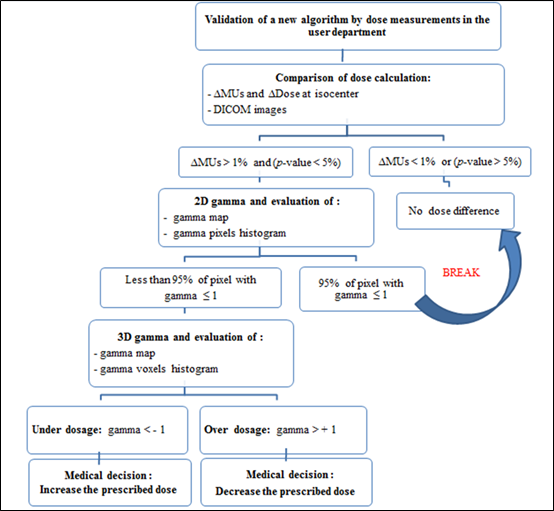FIG. 1: Summary of the method for making a medical decision.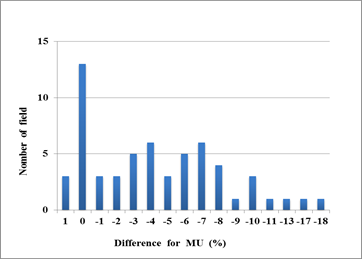FIG. 2: The number of beam as a function of percentage difference in MUs for 59 fields. The differences for MUs were calculated according to Equation 1. We note that using test algorithm in plan 2 compared to reference algorithm in plan 1, the MUs were not changed for 13 fields, but the MUs were lower for 43 fields and higher for 3 fields.

The principle of the method described below is illustrated in Figure 1. In a first step the difference in MUs, the dose at isocenter and p-value must be calculated. This allows the transfer of actual delivered dose (instead of the prescribed dose) from one treatment calculation to another, providing other variables remain unchanged. In this way the consequences of a change in the method of calculation can be readily made clear to the radiation oncologist. If the difference in MUs is more than 1% with p-value < 5%, the 2D gamma will be quickly proceeded as a second possibility to compare the dose distribution. The 2D global gamma index shows the dose difference in a plane. Using a 2D gamma evaluation the gamma map and the gamma pixel histogram are calculated. If there is any fraction of the pixels with gamma >1 located in the region of interest (PTVs or OARs), then a 3D gamma requiring much longer computing time must be performed to estimate the under and over dosage resulting from one algorithm compared to the other. The overall 3D gamma index gives the dose difference in the volumes. Gamma maps provide a useful tool for interpreting gamma distribution and the location of the difference in PTVs or OARs. Gamma values can be displayed in various color codes in 2D or 3D gamma maps. The positive and negative difference values show the over and under estimated doses for PTVs and OARs to be identified.

Results

Delivered dose
MUs

The algorithm PBC-MB in plan 2 calculated significantly less MUs than reference algorithm in plan 1 by on average 5% (4.4 SD ; p < 0.001) with CL =13.6. Figure 2 shows the number of the beams as a function of percentage difference in MUs between reference and test algorithms. PBC-MB applied to plan 2, yielded a lower number of MUs compared to plan 1 using PBC algorithm. Considering these results the use of plan 2 instead of plan 1 to treat the patients would result in under dosage to PTVs and a different dose distribution which may be better for the OARs. This difference may have a clinical impact compared to the reference plan. This overall difference of 5% is the main result of this study. However, different cancer sites should be considered individually before making any rule of dose modification.

Isocenter dose
The test algorithm in plan 3 calculated significantly higher dose at the isocenter than the prescribed dose in plan 1 by on average 5.2% (1.4 SD, p = 0.028) with CL = 7.9. This means that using the MUs calculated for plan 1, in plan 3, produces a delivered dose that is higher by about 5% than the dose prescribed by the oncologist. For sites surrounded by parenchyma which have a very low density, from 0.2 to 0.4g/cm3, the dose normalization method using MUs from plan 1 to calculate the dose in plan 3 using test algorithm confirms that the dose delivered was higher by 5% compared to the prescribed dose using reference algorithm.

Considering that plan 2 (PBC-MB) is more realistic, because it take into account the heterogeneity correction, we can give the following example: if the oncologist’s prescription was 66Gy using reference algorithm, a dose higher by 5% (close to 69Gy) would have been “actually delivered” with the corresponding clinical result. However, using PBC-MB, the oncologist’s prescription of 66Gy would “really” give 66Gy to the patient, once again with the corresponding clinical result, which may be expected to be reduced compared to that with plan 1. So, using PBC-MB without modifying the prescribed dose will result in a significantly lower effective dose delivered to the patient compared to that in the original plan using reference algorithm. However, a lower dose to both PTVs and OARs may result in an overall better outcome and may give a better result according to the respective positions of tumor control probability and normal tissues complication probability curves.19

Dose distribution in 2D and 3D
2D Gamma

The 2D gamma was calculated considering all pixels for all patients using only the transverse plane through isocenter for plan 1 and plan 2. The results for the 2D gamma show the pixels where the gamma > 1 using the set criteria (3%, 3 mm) in the comparison of the two algorithms. Comparing plan 1 and plan 2 for all patient (n = 6), the maximum gamma value was 2.5, average gamma value of 0.3 (2.2 SD) and CL = 4.61, depending on the site location. The maximum gamma value (2.5) was observed for the parenchyma site. The gamma evaluation shows that the difference in the dose between the two algorithms could be as large as ±8% in low gradient high dose areas.

Figure 3 (a) shows a sample of a gamma map in 2D in the traverse plane from the comparison between the two algorithms. This includes two PTVs located in a parenchyma tumor using plan 1 and plan 2. The gamma map was calculated in 2D using DICOM images including the PTVs, healthy lung and spinal cord. The red shading indicates that gamma values were above unity (out of tolerance). Figure 3 (b), shows the CT scan for the parenchyma site and the red circle indicates the PTV2 and the yellow circle the PTV1. Each PTV was treated using 3 fields. The comparison between the gamma map (Figure 3 (a)) and the CT scan (Figure 3 (b)) is obvious, it yields colored patches in red (gamma >1) located in PTVs and OARs.

3D Gamma
The comparison between reference algorithm vs. test algorithm using gamma (3%, 3 mm), showed in all patient cases an under estimating dose for PTVs and over estimating dose for OARs when plan 2 was used. There are also a significant difference between both GVHs (Dt > Dr) and (Dt < Dr), p < 0.05. Figure 5 shows a sample of the 3D gamma map resulting from the comparison between the two algorithms for a parenchyma tumor combining plan 1 and plan 2. The results shows that using test algorithm the dose for OARs was higher (red coloring), but a fraction of the PTVs will be under dosage (blue color) compared to reference algorithm.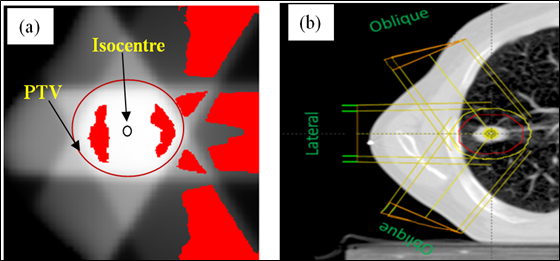FIG. 3: A sample gamma map in 2D plotted on the transversal plane for comparing the test and reference algorithms in plan 1 and plan 2. The red coloring in graph 3 (a) indicates that gamma values were > 1. The circle landmarks in Figure 3 (a) indicate the PTV contours around the isocenter point in the transverse plane. On the right, Figure 3(b) shows the CT scan for the parenchyma site; the red circle shows PTV2 and the yellow circle shows PTV1.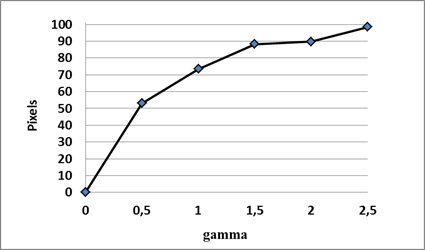FIG. 4: The cumulative gamma pixel histograms in 2D obtained when comparing the two algorithms for the 6 fields used to irradiate PTV1 and PTV2 located in the parenchyma site. We note that the gamma index criterion: 95% of pixels with gamma index ≤ 1 was not satisfied.

Figure 4 shows the gamma pixel histograms in 2D obtained from the comparison between the two algorithms for 6 fields used to irradiate the PTV1 and PTV2 located in the parenchyma site. In this case we can see that only 75% of pixels have a gamma index ≤ 1 using (3%, 3 mm). The tolerance of 95% pixels with gamma ≤ 1 was not satisfied.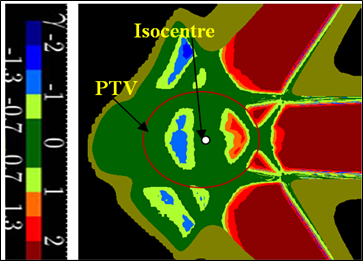FIG. 5: A sample gamma map in 3D plotted in the transverse plane for comparing test and reference algorithms for the parenchyma site including 6 fields. The red and blue coloring indicates that gamma is outside the tolerance with over or under estimation of the dose respectively. Khaki coloring indicates the pixels with doses lower than 10% of the prescribed dose. Black coloring indicates the pixels outside the patient. The circle landmarks in the figure indicate the PTVs contours around the isocenter point in the transverse plane.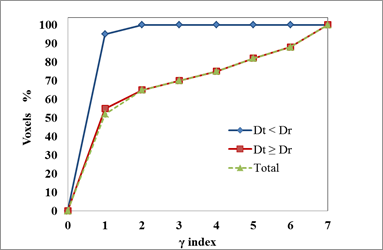FIG 6: Cumulative GVHs in 3D obtained when comparing the two algorithms for 6 fields used for parenchyma site. It can be seen that 5% of the voxels having γ > 1, which indicates an over dosage. Conversely for the under dosage, 45% of the voxel have a (γ < -1). This means that the tolerance level, 95% of voxels having gamma ≤ 1, is not obtained using PBC-MB method for plan 2.

Figure 6 shows the absolute values of the cumulative GVHs in 3D. The GVHs separate the total voxels into positive and negative voxels for the comparison of both algorithm which discriminate the under and over dosage regions. It can be seen that 5% of the voxels having an over dosage (γ > 1) deviates from the tolerance, which reaches the level of 95%. Conversely for the under dosage voxels, 45% of the voxel have a (γ < -1). It should be noted that this is a relative evaluation within two groups of voxels (positives vs negatives) and does not give absolute information. It must be estimated for the spatial distribution of colored areas in Figure 5.

Discussion

2D and 3D gamma evaluations
In this paper we describe a method based on the gamma function (2D and/or 3D) to evaluate the dose differences and it is applied to a change from PBC with no density correction to PBC with modified Batho’s method. The 2D and 3D gamma analysis based on plan 1 and plan 2 for each patient using all treatment fields. The gamma values reflect changes in the relative dose distribution in the PTVs and OARs between plan 1 and plan 2. In this study plan 2 and plan 3 were used to carry out the quantitative evaluation. After the validation of the newer algorithm in our department, the ICRU recommendation, were used to validate plan 2. Plan 3 was only to compare the prescribed dose. The gamma analysis showed a disagreement between the two dose distributions.

The average value for gamma in 2D was less than 1 for all patients in a given treatment plan, but the maximum can be up to 2. The comparison of GVHs in 3D allows qualifying this difference (under or over dosage) and demonstrated an under-dosage using test algorithm compared to reference algorithm. The importance of gamma maps is that showing the location of area which does not respect the tolerance levels in the CT-scan for the patient. The 3D gamma maps showed underestimating dosage (blue color) located in the PTVs and overestimating dosage for OARs (red coloring) for all patients. However to take into account the heterogeneity correction for lung, using (plan 2), the dose calculated to the OARs located beyond the tumor will increase due to attenuation correction. In this case the gamma index will fail but the dose to the OARs will be closer to reality. This means that using tolerance level (3%, 3 mm) for the gamma passing rate showed a dose difference in critical anatomic region around the PTVs, as showing in Figures 3 and 5. This is an alert for the physicist and the oncologist that the gamma passing rate for OARs is not satisfied (gamma >1). However in good practice the final verification using dose volume histogram (DVH) allows to check the satisfaction of the protective constrains for the OARs before the final validation of the treatment. Chaikh et al showed that the PBC-MB calculated a higher mean dose for normal lung, the dosimetric parameters derived from DVH were higher 8% for OARs.20

Tolerance levels to compare dose calculation algorithms in clinical use
As mentioned above, the first steps in the method in Figure 1 (determining the change in MUs, or the change in isocenter dose with the new MUs) are standard methods that are routinely used. Then the second approach is the use of 2D and 3D gamma maps and histograms. The comparison of dose calculation algorithms have one very important particularity, it is that the dose difference is only related to the change of calculation method. This means, turning on the modified Batho’s method, has not moved any beam edges, energy or angle. It is interesting to note that, the average γ values for all patient (n = 6) were less than the unity when using a 2D gamma evaluation with (3%, 3 mm) criterion. On a (3%, 3 mm) 2D gamma, all points would have a γ < 1, and so the flow chart on Figure 1 would conclude "no dose difference" although we observed a prescriptions dose difference higher than 3%. The histogram pixels gamma and GVHs presented in Figures 4 and 6 showed that the 95% of pixels/voxels had γ< 1 not respected for all patient (n = 6). Similarly if a change in algorithm made all beam edges move out by just under 3 mm, this would again pass the 2D gamma, rather than alerting the physicist to the very serious change. Whilst the gamma index is a very useful tool for comparing measured and calculated dose differences, in situations where the measurement uncertainties introduce a mix of positional and dosimetric uncertainties, its utility for comparing the results of two planning algorithms is less obvious. A better tool in this case would be a simple dose difference map. A consideration of how much of the area exceeds 1%, 2% or 3% would be a better measure than the gamma index as used here (3%, 3 mm). Consequently, to compare dose calculation algorithms for the same patient and the same configuration it would be better to use more sensitive criteria for dose difference for gamma evaluation. It is also possible to imagine some future algorithm change that uniformly changes the dose at every point by 2.99%. This could have very different consequences according to the local level of dose since radiobiological responses follow sigmoid curves.19 We recommend that the tolerance level to compare two algorithms should be adapted and defined for clinical use for the various tests.

The need to adjust the prescribed dose in radiotherapy
Clinicians should not forget that the visualized dose distributions obtained from TPS are only approximate representations of the real dose distributions. Thus, when changing from one algorithm to another in a radiation oncology department, modifications in the dose calculation must be carefully identified. It has been recently suggested that there is a need to adjust the prescribed dose of radiation especially after the implementation of the Anisotropic Analytical Algorithm (AAA) in the clinic.21 However, in our department this question was raised with the transition from a Clarkson algorithm to a PBC algorithm without heterogeneity correction.22 In fact the difference between MUs calculated using the Clarkson algorithm and PBC without heterogeneity correction was less than 1% for lung sites with a SD smaller than 1%. Subsequently we investigated the transition from PBC without heterogeneity correction (reference algorithm) to PBC-MB (test algorithm). The differences found for very heterogeneous volumes were then clearly larger, and truly raised the question of an objective decision process to change our prescription habit in order to maintain the same clinical results.

Our comparison, based on 59 fields, demonstrated that the calculated dose in MUs using the test algorithm was less than with reference algorithm. On average, deviations as large as 5% exist between the two algorithms. The differences in MUs were influenced by the field size, the anatomical structures surrounding the lung and the beam intensity. Thus, for beams with a long path through the lung the difference in MUs was increased. A statistical evaluation based on Wilcoxon’s test showed that this difference between MUs was significant. The deviations between the two algorithms were very significant for cancer located in lung parenchyma. The significant difference in MUs reflects the under-dosage observed in 3D gamma when test algorithm was used. Consequently, the risk due to the change in the algorithm from PBC to PBC-MB was a reduction in the dose delivered in MUs to the PTVs. Therefore, when changing from algorithm PBC to PBC-MB, a routine adjustment of the prescription made by the radiation oncologists appears necessary. In terms of the results found in the present study, this adjustment could be an increase of +5% in the prescribed dose in Gy, in order to continue to deliver the same actual dose to the tumor as when using the reference algorithm.

Instead of deciding to alter our prescription habits according to a certain level of difference in dose, it appeared to us more reliable to statistically ascertain the observed differences. The choice of an alpha risk of 5% appeared natural to us as it is used for almost all the statistical decisions in medico-biological sciences. Therefore we recommend testing and evaluating the new calculation algorithms for different anatomical sites and especially those where strong differences in tissue densities exist, as in thoracic locations. These results, if significant, should be discussed among clinicians and medical physicists to decide whether or not an alteration of the current prescription procedure should be considered.

Limits of this study
The main limitation of this study was the number of patients. However, the use of the Wilcoxon's test allowed us to calculate p-values for a small population size.17 In fact, the comparison of PBC and PBC-MB algorithms doesn’t give the present state of the art. For example, there is more accurate algorithm such as AAA and Collapsed Cone Convolution superposition algorithm.23, 24, 25, 26 But, the PBC with and without heterogeneity corrections were available algorithms in our department. These algorithms were simply used as an example to show the application of a new comparative technique and to validate our method. However, this method is general and can be used to compare any other algorithm in present and future practice.

The confidence limit is based on the average deviation between dose calculation using the reference and test algorithm and the SD. The multiplicative factor of 1.96 has been proposed by Palta et al.18 for having 5% of the individual points exceeding the tolerance level. In this study we observed higher values to CL related to higher values for SD. The large standard deviations for ∆MUs and ∆Dose at the isocenter are due to the heterogeneity of our population. Further studies that include patients presenting the same type of tumor site could certainly refine our conclusions.

A comparison of MUs proved to be useful for the first step comparison of algorithms. However the comparison of two different techniques of treatment is not possible by only comparing MUs since other parameters change besides the calculation method. For this reason, the 2D or 3D gamma analysis methods are convenient to compare techniques (i.e., IMRT or VMAT vs RT3D) since they just require DICOM files of two dose distributions, whatever their origins, for the two treatment plans and do not need the MUs. Moreover, the 3D gamma analysis shows the over or under estimation dose areas. However, 3D gamma takes much longer to carry out than 2D gamma. To accelerate the 3D gamma calculation the low dose levels, for instance below 10%, could be eliminated from the comparison.16 The gamma evaluation could have false positive and or negative and the common criterion (3%, 3 mm) is insufficient for patient IMRT quality assurance.27, 28

Conclusion

In this study we introduced a global method based on MUs and gamma evaluation in 2D and 3D to adjust the prescribed dose in radiotherapy. This method enables physicians and the oncologists to be aware of treatment modifications associated with a change in dose calculation software. The 2D and 3D gamma evaluation confirms the under dosage observed with PBC-MB using MUs comparison. For lung tumors, according to this study, the prescribed dose had to be adjusted by 5% when moving from PBC algorithm to PBC-MB in order to obtain the same delivered dose in the PTVs and the same clinical results as for the former reference treatment plan.

Acknowledgement

We thank Dr Alison Foote (Grenoble Clinical Research Center) critically reading our manuscript and editing the English.

Conflict of Interest

The authors declare that they have no conflicts of interest. The authors alone are responsible for the content and writing of the paper.

Received August 19, 2014; Revised October 06, 2014; Accepted October 06, 2014; Published Online October 13, 2014

Corresponding author: Abdulhamid Chaikh; Department of Radiation Oncology and Medical physics, Grenoble University Hospital, Grenoble, France.